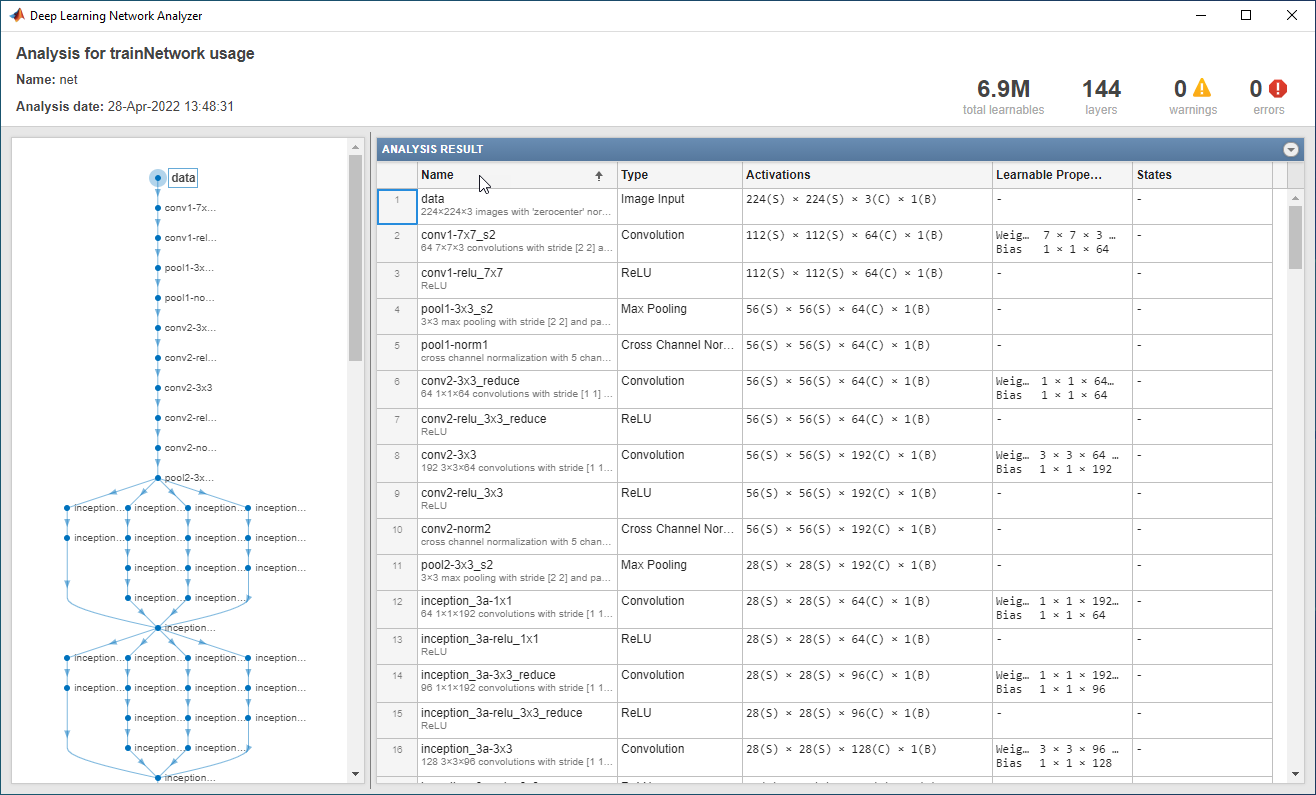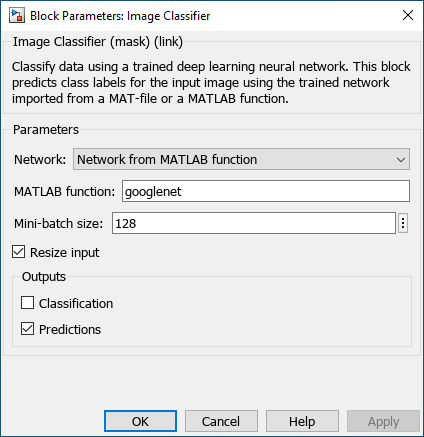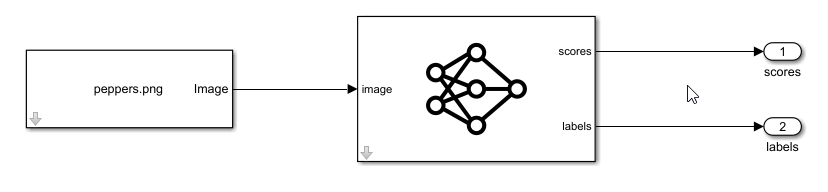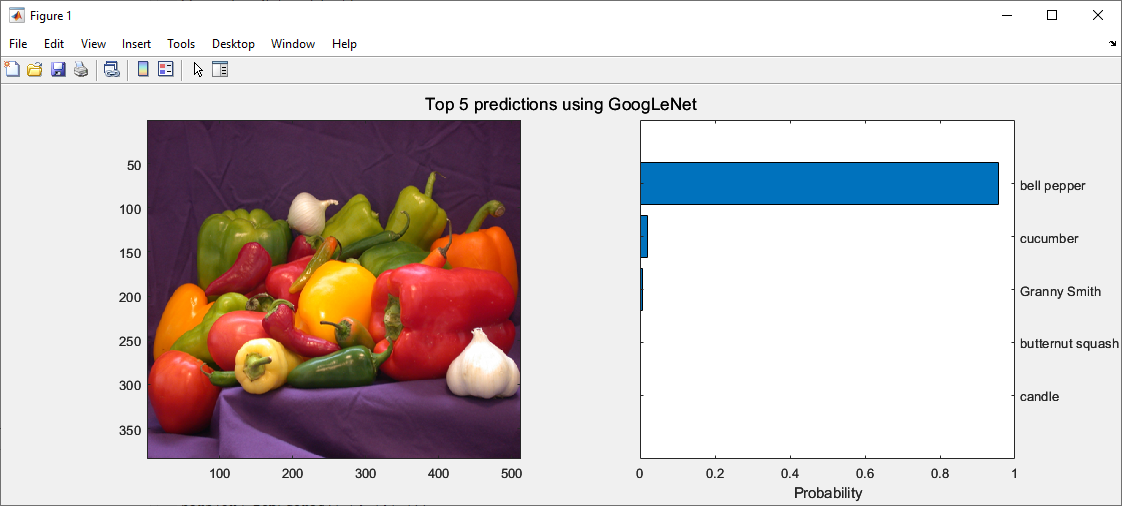## Generate Code for Blocks from Deep Neural Networks Library

You can generate optimized code for Simulink® models containing a variety of trained deep learning networks. You can implement the deep learning functionality in Simulink by using MATLAB® Function blocks or by using blocks from the Deep Neural Networks library. For information on the blocks in the Deep Neural Networks library, see Blocks (Deep Learning Toolbox).

You can configure the code generator to take advantage of the Intel® Math Kernel library for Deep Neural Networks (MKL-DNN). You can also generate generic C or C++ code that does not depend on third-party libraries. The generated code implements the deep convolutional neural network (CNN) by using the architecture, the layers, and parameters that you specify in network object.

### Classify Images by Using GoogLeNet

GoogLeNet has been trained on over a million images and can classify images into 1000 object categories (such as a keyboard, coffee mug, pencil, and animals). The network takes an image as input, and then outputs a label for the object in the image with the probabilities for each of the object categories. This example shows how to perform simulation and generate C++ code for the pretrained `googlenet` deep convolutional neural network and classify an image. The pretrained networks are available as support packages from the Deep Learning Toolbox™.

1. Load the pretrained GoogLeNet network. You can choose to load a different pretrained network for image classification. This function requires the Deep Learning Toolbox Model for GoogLeNet Network support package. If this support package is not installed, then the function provides a download link.

`net = googlenet;`

2. The object `net` contains the `DAGNetwork` object. Use the function to display an interactive visualization of the network architecture, to detect errors and issues in the network, and to display detailed information about the network layers. The layer information includes the sizes of layer activations and learnable parameters, the total number of learnable parameters, and the sizes of state parameters of recurrent layers.

`analyzeNetwork(net);`3. The image that you want to classify must have the same size as the input size of the network. For GoogLeNet, the size of the `imageInputLayer` is 224-by-224-by-3. The `Classes` property of the output `classificationLayer` contains the names the names of the classes learned by the network. View 10 random class names out of the total of 1000.

```classNames = net.Layers(end).Classes; numClasses = numel(classNames); disp(classNames(randperm(numClasses,10)))```
``` speedboat window screen isopod wooden spoon lipstick drake hyena dumbbell strawberry custard apple```

2. Add an Image From File block from the Computer Vision Toolbox™ library and verify that the `File name` parameter to `peppers.png`.

3. Insert an Image Classifier block from the Deep Learning Toolbox > Deep Neural Networks library. Set these block configuration parameters:

• Set the Network parameter to ```Network from MATLAB function```.

• Set the MATLAB function parameter to `googlenet`.

• Select the Resize input parameter.

• Select the Predictions parameter.

• Clear the Classifications parameter.4. Add two Outport blocks and connect them to the Image Classifier block. Connect the Image Classifier block to the Image From File block.5. Save the model as `googlenetModel.slx`.

### Simulate Model

1. Open the Configuration Parameters dialog box. Select the Solver pane. Set the Type parameter to `Fixed-step`. This setting maintains a constant (fixed) step size, which is required for code generation.

2. Select the Simulation Target pane. Set the Language to `C++`.

3. On the Simulation Target pane, verify that the Target Library parameter in the Deep learning group is set to `MKL-DNN`.

4. Click OK to save and close the Configuration Parameters dialog box.

5. To build and simulate the model, use this command:

`out = sim('googlenetModel');`

6. Display the top five predicted labels and their associated probabilities as a histogram. Because the network classifies images into so many object categories, and many categories are similar, it is common to consider the top-five accuracy when evaluating networks. The network classifies the image as a bell pepper with a high probability.

```im = imread('peppers.png'); predict_scores = out.yout{1}.Values.Data(:,:,1); [scores,indx] = sort(predict_scores,'descend'); topScores = scores(1:5); classNamesTop = classNames(indx(1:5)) h = figure; h.Position(3) = 2*h.Position(3); ax1 = subplot(1,2,1); ax2 = subplot(1,2,2); image(ax1,im); barh(ax2,topScores(1,5:-1:1,1)) xlabel(ax2,'Probability') yticklabels(ax2,classNamesTop(5:-1:1)) ax2.YAxisLocation = 'right'; sgtitle('Top 5 predictions using GoogLeNet') ```### Generate Code

1. Open the Simulink Coder or Embedded Coder app. The C Code tab opens.

2. In the Configuration Parameters dialog box, if you have a Simulink Coder™ license, set the System target file to `grt.tlc`. If you have Embedded Coder®, set the System target file parameter to `ert.tlc`.

3. Set the Language to `C++`.

4. Select Generate code only.

5. On the Code Generation > Interface pane, set the Target Library in the Deep learning group to `MKL-DNN`.

6. On the Interface pane, clear the MAT-file logging parameter.

7. If the System target file parameter is set to `ert.tlc`, on the Interface pane, select the variable-size signals parameter.

8. Click OK to save and close the Configuration Parameters dialog box.

9. On the C++ Code app, click Build.